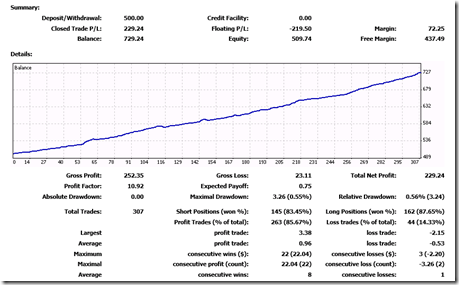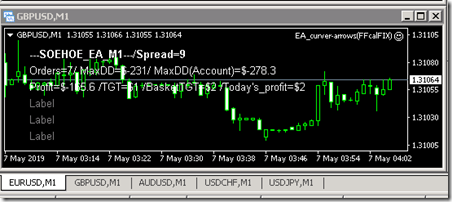# FT for EA_curve arrow

This is FT result.I am running 5 major pair. TF is M1Max DD (floating ) is \$270

Set file.

Note=M1
MAGIC=1
TXT_left_Margin=30
fontsize=10
Slippage=3
Reverse_Mode=false
Max_order=3
Use_Order_distance_Pips=true
Order_distance_Pips=5
Note2=— Money management —
MM=1
FixLotSize=0.01
Max_martingale=5
Multiplier=2.0
Risk=5.0
Note3=—Attack Martingale —Add multiple order every xx pips
Use_Attack_Martin=true
Martin_Lotsize=0
Martin_Multiplier=2.0
Max_Martin=5
Use_AUTO_PipStep=false
PipStep_default=10
Pip_Multiple=1.0
Martin_takeprofit=10
Martin_stoploss=0
Note4=— Profit dollars that EA close all positions (same MAGIC#)—
Profit_dollar=2
Profit_by_manual=0.0
Profit_by_equity_percentage=0.0
Note5=— Basket Profit dollars to close all charts positions —
Note6=— Equity guard (total balance)XX percent of equity-drawndown to close all positions—
XXpercent=0
Note7=— Daily profit Target dollars (EA will stop orders if reach to the target & restart in next day)—
Use_Daily_TGT=false
Daily_profit_TGT=0.0
After_TGT_reached=1
Note8=— Takeprofit & Stoploss —
takeprofit=0.0
stoploss=0.0
Opposite_close=false
Note9=–Trailing stop–
Use_trailingStop=false
TS_Start=20.0
TS_Stop=10.0
TS_Step=5.0
Note9a=–Break even (BE)–
Use_BE=false
BE_Start=5.0
MoveStopPips=0.0
Note10=–EA time filter –Stop or Run EA during the time period(hours)
UseTimeFilter=3
Start_Time=10
End_Time=18
Note11=—-Time based StopLoss—-Order should be closed after XXX hours.
UseTimeBasedStopLoss=false
TimeBasedSLhours=100
Note12=—NEWS Filter —Select impact to stop EA
Use_News_Filter=false
MinimumImpact=1
MinsBeforeNews=60
MinsAfterNews=60
OffSetHours=0
Note13=—Filter —
Filter_TF=60
Note14=—EMA filter —
Use_EMA_filter=false
period1=14
period2=26
Note15=—Stochastic filter —
Use_stochastic_filter=false
k_period=14
d_period=5
Note16=—Volatility filter —
Use_Volatility_filter=false
Reverse=false
MA_period=34
value=0.5
Note17=—RSI filter —
Use_RSI_filter=false
Period=14
Note18=—Friday Timer —Close all orders at xx:xx on Friday.
Use_FridayTimer=false
CloseTime=22:00
Stop_On_Friday=false
Note19=— Indicator settings —
SSP=6
CountBars=2250
SkyCh=13

### 2 件のコメント

•majid says:

hello dear tanaka.
this result is which account/broker/set.
i want run a 30-40 usd account.
i need you learn me how run this EA.
you still code free EA with free indicators?

•Tanaka Akiko says: